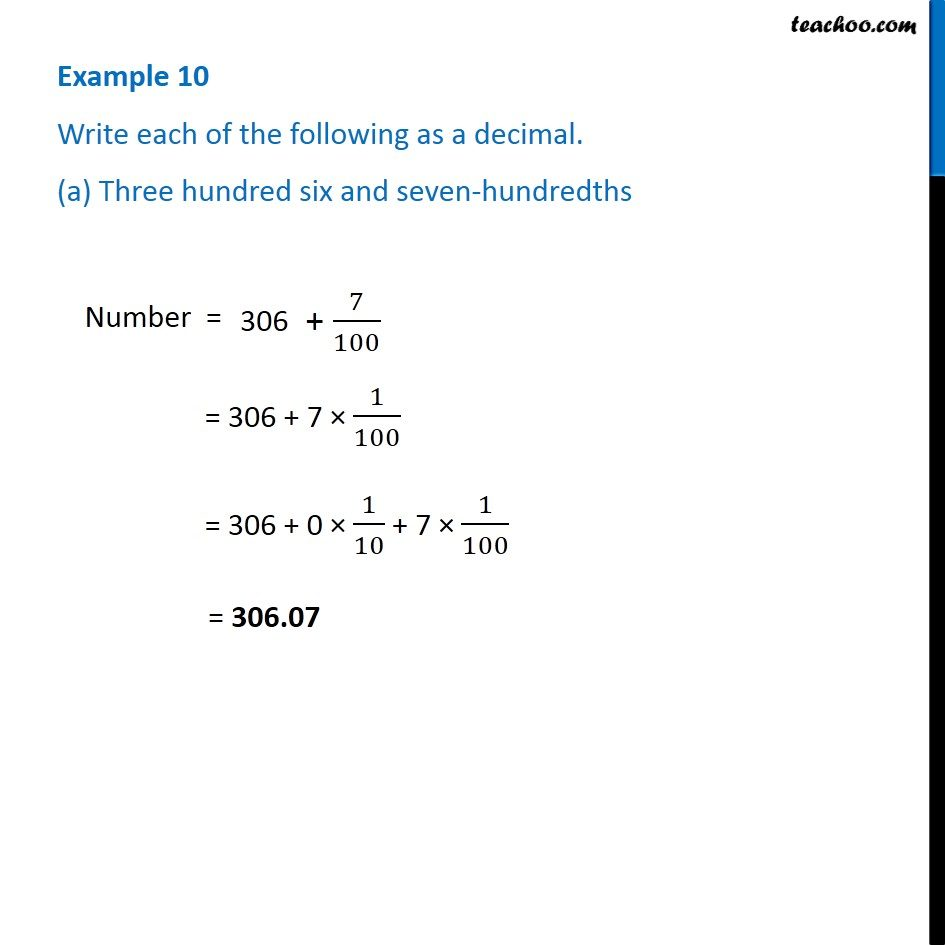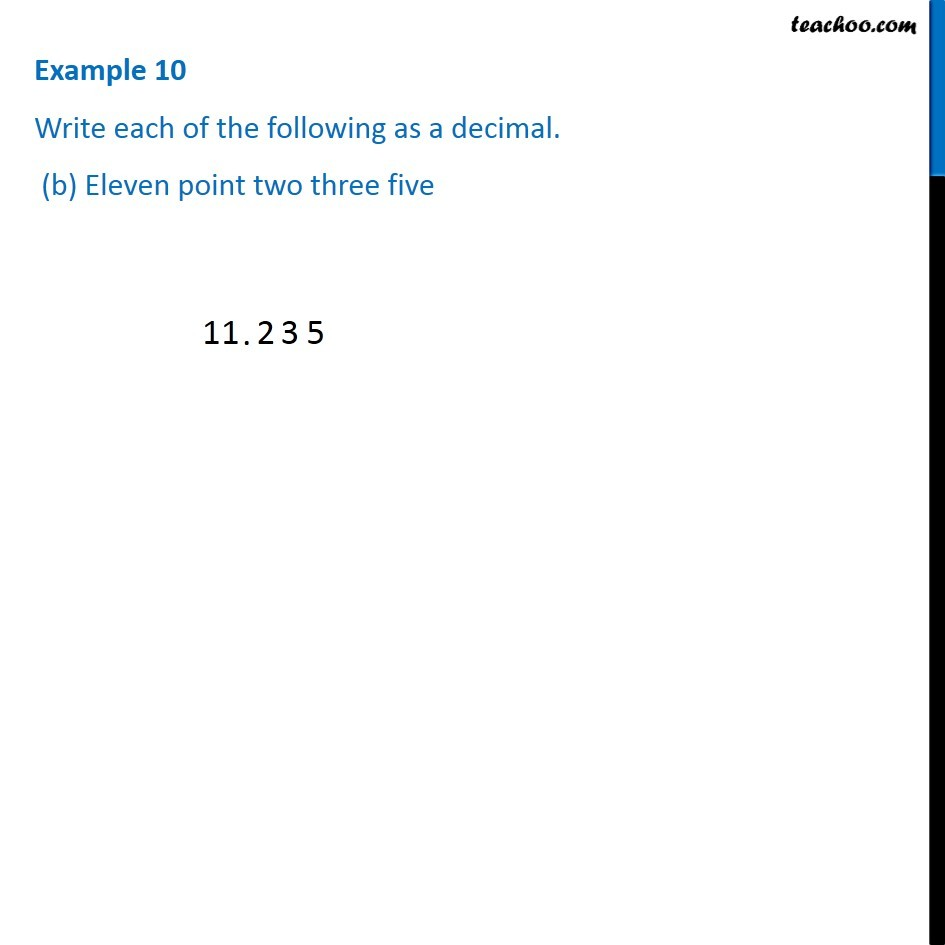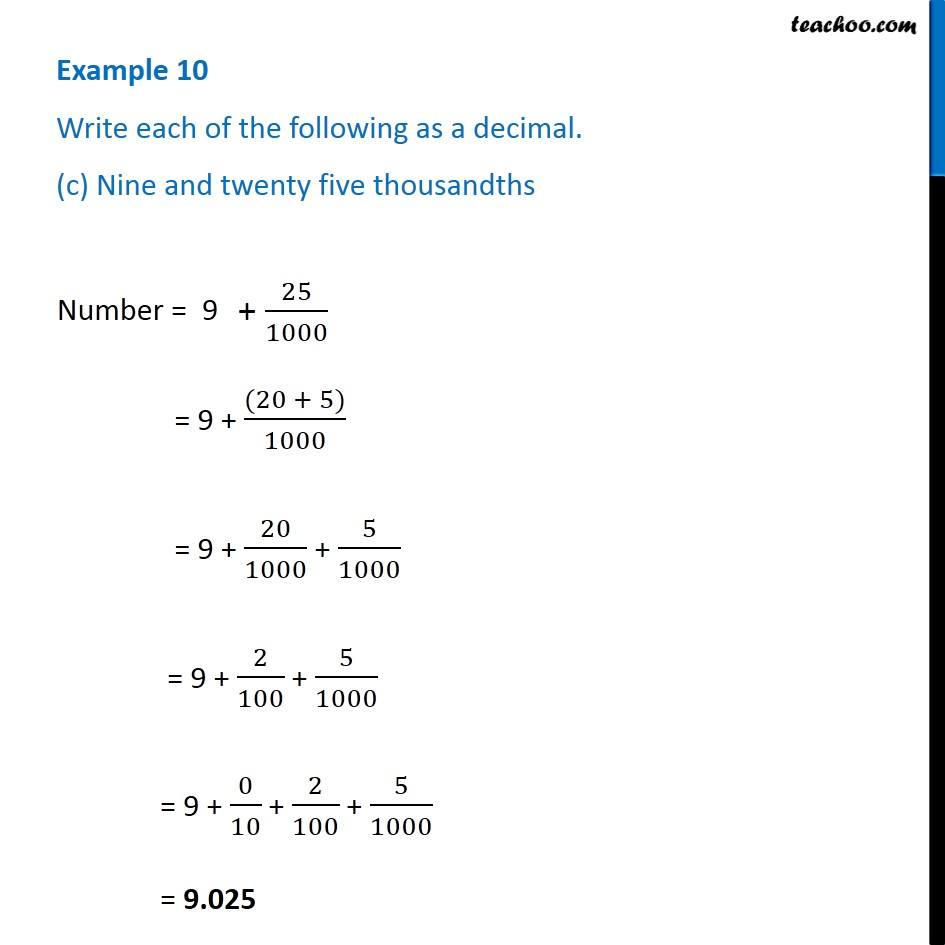Subscribe to our Youtube Channel - https://you.tube/teachoo

1. Chapter 8 Class 6 Decimals
2. Serial order wise
3. Examples

Transcript

Example 10 Write each of the following as a decimal. (a) Three hundred six and seven-hundredths Number = = 306 + 7 × 1/100 = 306 + 0 × 1/10 + 7 × 1/100 = 306.07 Example 10 Write each of the following as a decimal. (b) Eleven point two three fiveExample 10 Write each of the following as a decimal. (c) Nine and twenty five thousandthsNumber = = 9 + ((20 + 5))/1000 = 9 + 20/1000 + 5/1000 = 9 + 2/100 + 5/1000 = 9 + 0/10 + 2/100 + 5/1000 = 9.025

Examples

Chapter 8 Class 6 Decimals
Serial order wise